# Pandas Rename and Reorder Columns

Pandas has two ways to rename their Dataframe columns, first using the df.rename() function and second by using df.columns, which is the list representation of all the columns in dataframe. Let’s Start with a simple example of renaming the columns and then we will check the re-ordering and other actions we can perform using these functions

Renaming Columns

We have a dataframe of Students details and each of the column has a special character \$ prefixed to it’s columns and we now want to strip that special character from the column names

``````df = pd.DataFrame({'\$name':['Allan','Mike','Brenda','Holy'], '\$Age': [30,20,25,18],'\$Zodiac':['Aries','Leo','Virgo','Libra'],'\$Grade':['A','AB','B','AA'],'\$City':['Aura','Somerville','Boon','Gannon']})
``````We will replace the df.columns list value with the new list value by stripping the \$ character using the str.replace() function

``````df.columns = df.columns.str.replace('\$','')
``````

Let’s check the new column values after executing the above line of code

``````df.columns
Index(['Age', 'City', 'Grade', 'Zodiac', 'name'], dtype='object')
``````

The new column values doesn’t have those \$ character in the column namePandas rename column with list

You can just replace the existing column values with the new column in the list

``````df.columns = ['x','y','z','p','q']
df
``````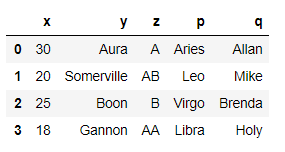Re-Order Columns

We will re-order the column by moving column z to second and y to third and swap p and q. Let’s see how we can do this by simply assigning the new values in a list to df.columns

``````# Re-order Columns
df = df[['x','z','y','q','p']]
df
``````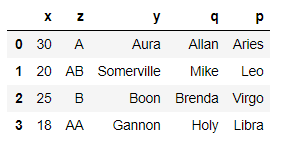Fill None columns using fillna

Let’s change one of the column to None in the above dataframe

``````df.columns = ['a','b',None,'d','e']
``````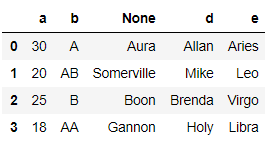The column c is replaced with None. Now if we want to replace the None Columns with any default values

We have replaced the None column with z using fillna

``````df.columns=df.columns.fillna('z')
df.columns

Index(['a', 'b', 'z', 'd', 'e'], dtype='object')
``````

Pandas rename single column

Rename single column e with new_e

``````df.columns=[it.replace('e','new_e') for it in df.columns]
``````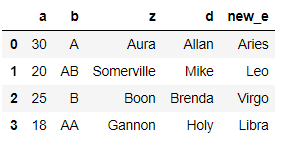We can also use the df.rename() function

``````df.rename(columns={'e':'new_e'},inplace=True)
``````

Pandas Rename Multiple Columns

We will now change the column z as new_z and d as new_d

``````df.columns = ['a','b','new_z','new_d','v']
``````

OR

``````df.rename(columns={'z':'new_z','d':'new_d'},inplace=True)
``````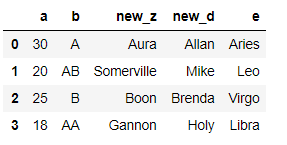Delete Column

Use the drop function to drop the selected column

``````df[df.columns.drop('d')]
``````

OR

``````df.drop(['d'],axis=1,inplace=True)
``````

Delete None Columns Name

Using the drop function just pass the None value to drop the Null Columns Name

``````df[df.columns.drop(None)]
``````

Rename Columns by Index

Here we are replacing the second column b with new_b

``````df.rename(columns={ df.columns: "new_b" })
``````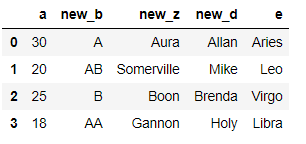Sort Columns by Values

Let’s create the new column values in non-alphabetical way as e,d,c,b,a

``````df.columns=['e','d','c','b','a']
``````Sort the above dataframe columns in Alphabetical way

``````df.columns=df.columns.sort_values()
df
``````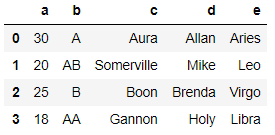Find Column difference between two dataframes

Let’s create two dataframe first and find out the columns difference between the two dataframe column values. The first dataframe has columns `[name,Age,Zodiac,Grade,City] and second one has column [name,Age,Favs,Grade,Interest]`

``````df = pd.DataFrame({'name':['Allan','Mike','Brenda','Holy'], 'Age': [30,20,25,18],'Zodiac':['Aries','Leo','Virgo','Libra'],'Grade':['A','AB','B','AA'],'City':['Aura','Somerville','Boon','Gannon']})

``````

Let’s find the differnce between the two columns names, Please note we are just find the column names difference and not the column values

``````df1.columns.difference(df.columns)
``````
``````Index(['Favs', 'Interest'], dtype='object')
``````

You can see the Above code gives us the two column names i.e. Favs and Interest which is not in the first dataframe

Conclusion

So we have seen how easy it is to Rename the column names and re-order the column values using df.rename and df.columns. There are lot of other useful functions within df.columns like equals, factorize, get_values etc. which can be used for any operations with the column names and values.

Tags:

Categories:

Updated: